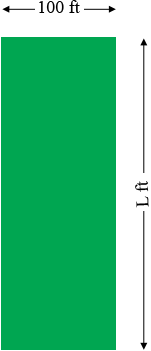SEARCH HOMEMath Central Quandaries & QueriesQuestion from Chad, a parent: I have 3 acres 100ft at the top and bottom what is the length of each side to make up the 3 acresThere are 43560 square feet in an acre so 3 acres would be $3 \times 43560 = 139590$ square feet. The area of a rectangle is the length times the width so if the length is L feet and the width is 100 feet then we have

$100 \times L = 139590$

and hence $L = 1395.9$ feet.

If the angles are not right angles the the plot might look likeoror many other shapes. Without knowledge of the size of the angles the distance from the top to the bottom can't be determined.

PennyMath Central is supported by the University of Regina and The Pacific Institute for the Mathematical Sciences.